# Investigate Mating Scenes

#### 2023-02-03

1. Introduction to mating scenes
2. Importing a dataset
3. Simulate a mating scene
4. Space
5. Time
6. Compatibility
7. Multi-population scenes
8. Multi-year scenes

# Introduction to mating scenes

This vignette consists of a brief introduction to the investigation of mating scenes using package mateable. A mating scene is a bout of mating where the coordinates of participating individuals are defined in one, two, or three dimensions: space, time, and compatibility. From such information we can quantify mating potential, the capacity for sexual reproduction based on the location, reproductive timing, and compatibility of prospective mates. Mating potential can be quantified for a pair of individuals based on the distance between them, the timing of their reproductive activity, and their compatibility. Similarly, mating potential can be defined for an individual within the context of the mating scene or for an entire scene.

library(mateable)
packageDescription("mateable")$Version ##  "0.3.2" oldpar <- par(no.readonly = TRUE) For any function in mateable you can learn more by typing a question mark before the function name, i.e. ?makeScene. To learn all the functions, type ?mateable and click index at the bottom of the page. # Importing a dataset To analyze data using mateable, you must convert a data frame containing spatial, temporal, and/or mating type information to a mating scene object. To do so, use the function makeScene, specifying which columns contain mating scene coordinates. In this section we look at flowering during 2012 in a remnant prairie population of Echinacea angustifolia, the narrow-leaved purple coneflower. The data frame ech2012 is included in the package. str(ech2012) ## 'data.frame': 53 obs. of 6 variables: ##$ tag     : num  12243 14583 15086 15128 17282 ...
##  $pop : chr "eelr" "eelr" "nwlf" "eelr" ... ##$ firstDay: Date, format: "2012-06-25" "2012-06-28" ...
##  $lastDay : Date, format: "2012-07-14" "2012-07-10" ... ##$ Ecoord  : num  1563.9 1384.3 18.1 1488.5 17.6 ...
##  $Ncoord : num 1559.5 1665.83 12.26 1609.33 1.26 ... This data set includes spatial and temporal information on all 53 plants that flowered in 2012 from the East Elk Lake Road and Northwest of Landfill populations. Each plant has a tag with a unique number for identification purposes and we listed the count of heads that the plant produced in 2012. The columns firstDay and lastDay indicate the first and last days that each plant produced pollen. Spatial coordinates are in meters. Note that some columns are integer and numeric. Columns firstDay and lastDay are saved in a Date format. makeScene can read some character formats. If you want to convert character or POSIX to the Date format, read about function as.Date or install package lubridate. For the first sections of this introduction to mateable we will focus on one population. eelr <- ech2012[ech2012$pop %in% 'eelr',]
eelr <- makeScene(eelr, startCol = "firstDay", endCol = "lastDay",
xCol = "Ecoord", yCol = "Ncoord", idCol = "tagNo")

Now, using other functions to produce visual and quantitative summaries of the data is simple.

We can make figures to plot the spatial and temporal dimensions of our mating scene. The figure on the right illustrates the reproductive period during 2012 for each of the 44 individuals in the population with a horizontal line starting on the date the individual first produced pollen and ending on the date when pollen was last produced. Here the individuals are sorted from bottom to top by their first day. The dots indicate the total number of individuals participating in mating on each day. Use R graphical parameters to change the look of the plot. We specify individuals to highlight by including their ID as an argument to the function parameter sub.

focalPlants <- c(17217, 17202, 14582, 15114, 7614, 1509, 17002, 7431, 3370)
par(mar = c(3,4,1,1), oma = c(2,0,0,0))
plotScene(eelr, c('s','t'), sub = focalPlants, N = 4, label.cex = 0.5, plot.lim.zoom = TRUE)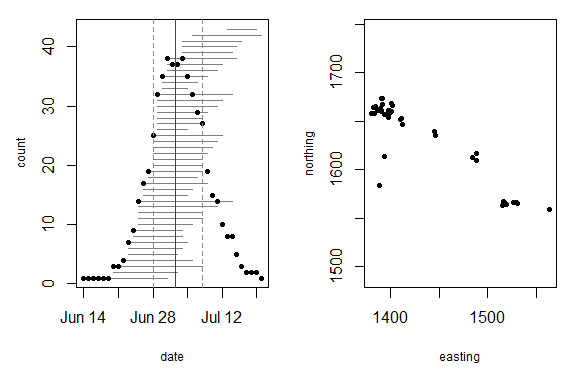Function matingSummary calculates many characteristics of the “whole scene.” We can save summaries as a new object eSum and look at characteristics of the entire mating scene, either by indexing or by name.

eSum <- matingSummary(eelr)

# Simulate a mating scene

Along with using data from real populations, we can also simulate scenes. Here we simulate a scene using values from the eelr summary as inputs for the simulation parameters.

# make scene based off eelr summary information
simScene <- simulateScene(size = nrow(eelr),
meanSD = eSum$meanSD, # mean start date sdSD = eSum$sdSD,  # standard deviation of start date
meanDur = eSum$meanDur, # mean duration of reproductive bout sdDur = eSum$sdDur, # standard deviation of duration of reproductive bout
xRange = c(eSum$minX, eSum$maxX), # range of spatial x-coordinates
yRange = c(eSum$minY, eSum$maxY)) # range of spatial y-coordinates

A simulated scene can be treated the same way as a scene made from real data, meaning all functions work in the same way. In addition, simulated scenes will always have all three dimensions (space, time, and compatibility), so you can examine any aspect of mating potential.

The coordinates in the spatial and temporal dimensions are generated from uniform and normal distributions, respectively. The coordinates in the compatibility dimension are, for each individual, two alleles selected at random from the number of total alleles in the scene (default is 10). In the third panel of the second figure allele shows the alleles for each individual; they are labeled 1, 2, 3, … , 10.

We can do a lot with a matingScene object. Let’s start by focusing on the spatial dimension of the mating scene.

# Space

Distance is a measure of isolation from mates. To characterize mating potential, which is inversely related to distance, we want proximity to mates. For this, we have function proximity.

eProx <- proximity(eelr, "maxPropSqrd")
eProx$pop ##  0.4925677 hist(eProx$ind$proximity, 30)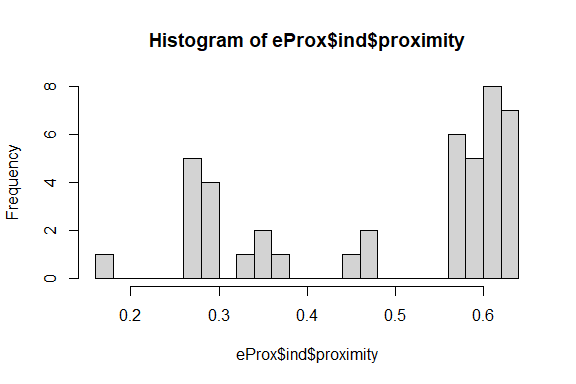Now that we have saved a proximity object, eProx, we can visualize it using function plotPotential. There are many ways to visualize it. The default returns three figures of pairwise values of proximity: a histogram of all pairs, a network diagram of a random subset of 9 individuals, and a heat map of all interactions between those same nine. In the network diagram, line width corresponds to the pairwise proximity of the individuals being connected, and the size of label indicates the individual’s mean proximity with all other individuals in the network. par(mfrow = c(1,3), oma = c(1,1,1,2), mar = c(4,3,1,3)) plotPotential(eProx) ##  "proximity"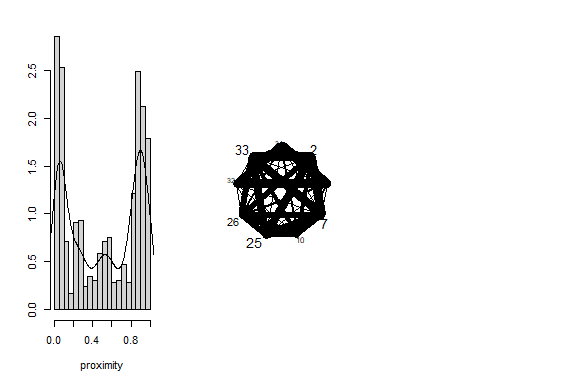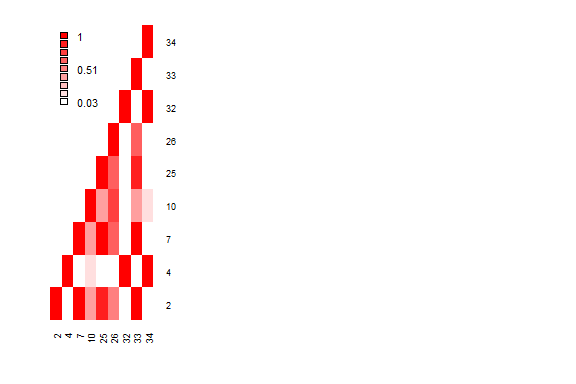If we want to focus on a particular subset of plants, then we can define them using the argument sub.ids. Here, we make only a network diagram of these focal plants, which we specify using plotType. par(mfrow = c(1,1)) plotPotential(eProx, plotType = "net", sub.ids = focalPlants) ##  "proximity"Notice plant 1509 is more isolated from potential mates than the other individuals. # Time Now we turn from the spatial dimension of the mating scene to the temporal dimension. We can visualize the coordinates of mating activity in time with a mating schedule. Just as we can calculate and visualize the spatial distances between all pairs using function pairDist, we can do the same in the temporal dimension with function overlap. Function overlap makes a matrix of overlapping days for all pairs and we can use a histogram to see the distribution. eOver <- overlap(eelr, compareToSelf = TRUE) # matrix of days overlapping hist(eOver, 40, main = "Histogram of days overlapping between pair")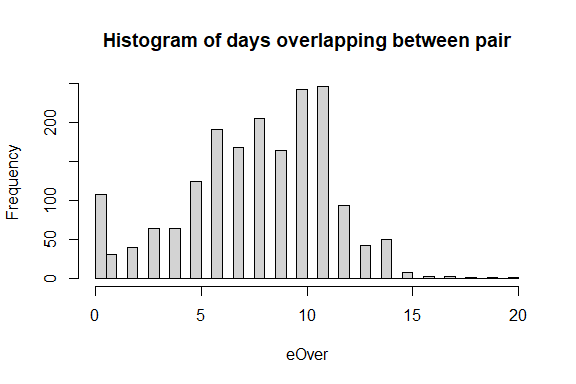We can look at the number of individuals participating in mating every day. eRecep <- receptivityByDay(eelr) # T/F receptive on each day str(eRecep) # matrix ## logi [1:43, 1:37] FALSE FALSE FALSE FALSE FALSE FALSE ... ## - attr(*, "dimnames")=List of 2 ## ..$ : chr [1:43] "1" "2" "3" "4" ...
##   ..$: chr [1:37] "1" "2" "3" "4" ... ## - attr(*, "origin")= Date[1:1], format: "2012-06-13" dailyReceptivitySummary <- receptivityByDay(eelr, summary = TRUE) dailyReceptivitySummary # a named integer vector ## 2012-06-14 2012-06-15 2012-06-16 2012-06-17 2012-06-18 2012-06-19 2012-06-20 ## 1 1 1 1 1 1 3 ## 2012-06-21 2012-06-22 2012-06-23 2012-06-24 2012-06-25 2012-06-26 2012-06-27 ## 3 4 7 9 14 17 19 ## 2012-06-28 2012-06-29 2012-06-30 2012-07-01 2012-07-02 2012-07-03 2012-07-04 ## 25 32 35 38 37 37 38 ## 2012-07-05 2012-07-06 2012-07-07 2012-07-08 2012-07-09 2012-07-10 2012-07-11 ## 35 32 29 27 19 15 14 ## 2012-07-12 2012-07-13 2012-07-14 2012-07-15 2012-07-16 2012-07-17 2012-07-18 ## 10 8 8 5 3 2 2 ## 2012-07-19 2012-07-20 ## 2 1 plot(as.Date(names(dailyReceptivitySummary)), dailyReceptivitySummary, xlab = 'date', ylab = 'count of receptive individuals')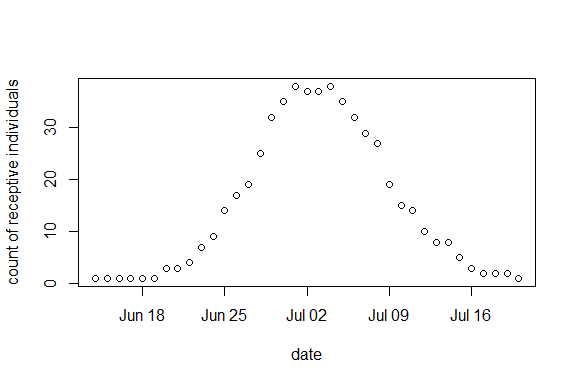Folks have calculated synchrony many ways for individuals, pairs, and populations. Our function synchrony does it all; well, it does a lot. We can specify many different synchrony measures, with enticing names, such as these (read the help for details): “augspurger”, “kempenaers”, “sync_prop”, “overlap”, “sync_nn”, “simple1”, “simple2”, and “simple3.” Also, we can calculate measures for different subjects: individual, pairs, and the whole scene (population). Here, for example, we calculate synchrony for all subjects using the overlap method and save it as eSync. Then we show the mean and median population values in red and blue, respectively. eSync <- synchrony(eelr, "overlap") hist(eSync$ind[, 2], 30)
abline(v = eSync$pop, col ="red", lwd = 2) abline(v = synchrony(eelr, "overlap", averageType = "median")$pop,
col = "blue", lwd = 2)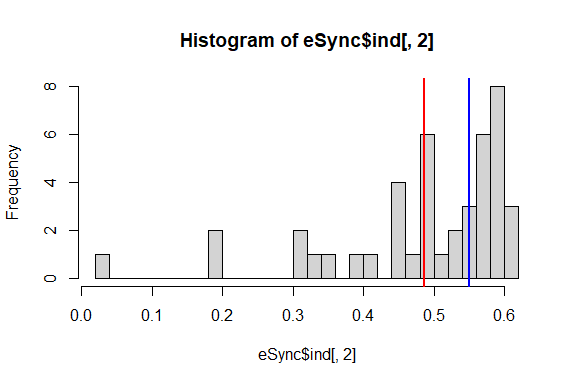Individual overlap indicates the proportion of potentital mates in the scene that were flowering averaged over all days that the focal individual participated in mating.

Just like we visualized spatial mating potential, we can visualize mating potential in the temporal dimension. Here we emphasize the same focal plants as we did above.

par(mfrow = c(1,3), mar = c(2,4,2,4), oma = c(4,4,4,4))
plotPotential(eSync, sub.ids = focalPlants)
##  "synchrony"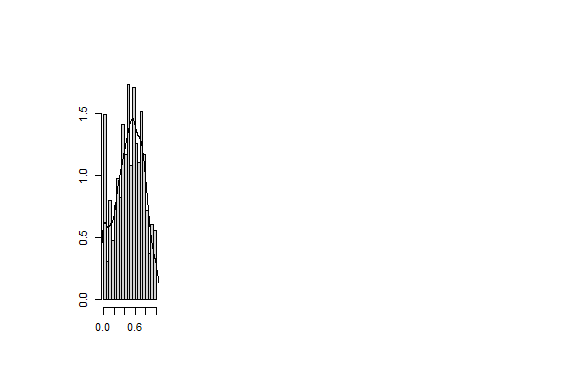Notice that 1509 is not isolated in time from potential mates.

We can use the function plot3DPotential to visualize multiple dimensions of mating potential.

par(mar = c(4,4,1,1))
plot3DPotential(list(eSync,eProx), sub.ids = focalPlants)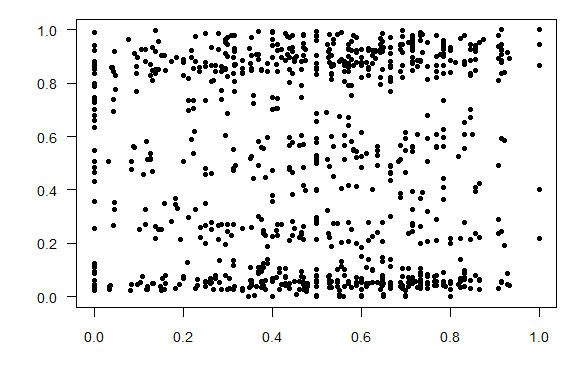# Compatibility

In animals, females are compatible mates with males but they are not compatible with other females. Similarly, males are only compatible with females. We say that mating potential between a pair of males is zero, between a pair of females is zero, and between a mixed-sex pair it is 1. Most animal species, though not all, have a breeding system like this. It’s more complicated in plants. Plants have many breeding systems, including self-compatibility and dioecy–dioecy is just like the simple case in animals. About half of all plants species have some kind of self-incompatibility system which makes it possible for some pairs to be mating incompatible, i.e. have mating potential of zero. Package mateable allows us to model the animal breeding system and the breeding system in Echinacea (sporophytic self-incompatibility). In the following examples, we show examples of each. We intend to add capability for handling more breeding systems in future releases of mateable.

Mating potentials in space and time are continuous because space and time are continuous. In contrast, mating compatibility between two individuals, as we have modeled it here, is either possible or not. Therefore mating potential for a pair is either 1 or 0.

sComp <- compatibility(simScene, "si_echinacea")
par(mfrow = c(1,3))
plotPotential(sComp, density = FALSE)
##  "compatibility"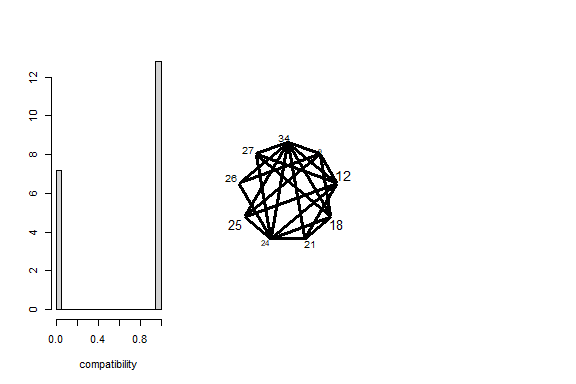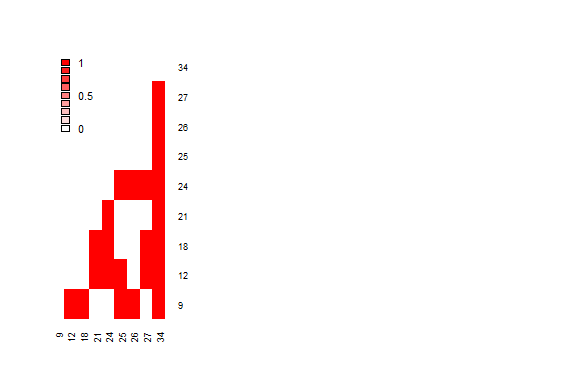par(mfrow = c(1,2))
plotPotential(sComp, plotType = c('net','heat'), density = FALSE)
##  "compatibility"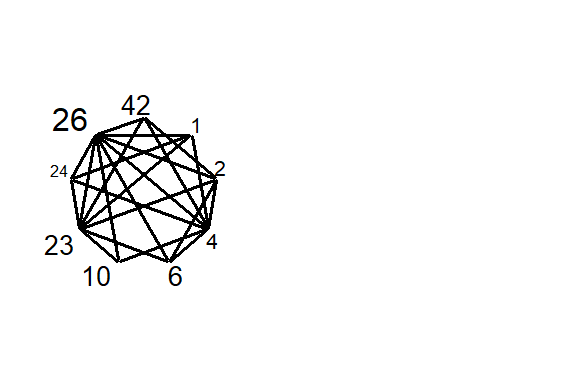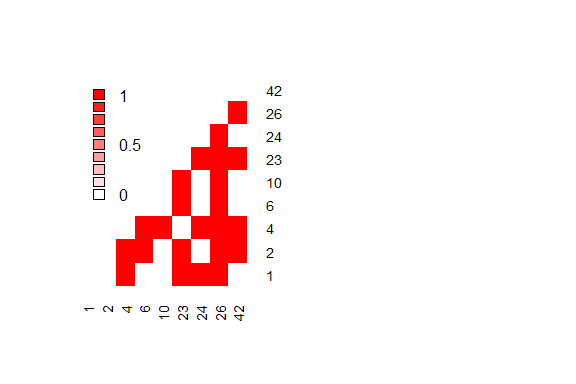# Multi-population scenes

Researchers may be interested in comparing two populations. There are several options for visualizing multiple populations in mateable. The dataframe ech2012 is included in mateable, which includes spatial and temporal data from two spatially isolated populations of Echinacea angustifolia.

Use the argument split when making a scene to create separate scenes delineated the values of the column specified in split:

ech2012a <- makeScene(ech2012, startCol = "firstDay", endCol = "lastDay",
xCol = "eCoord", yCol = "nCoord", idCol = "tag",
split = "pop")

par(mar = c(3,4,1,1), oma = c(2,0,0,0))
plotScene(ech2012a, plot.lim.zoom = TRUE) # spatial plot limits set by range of coordinates in each scene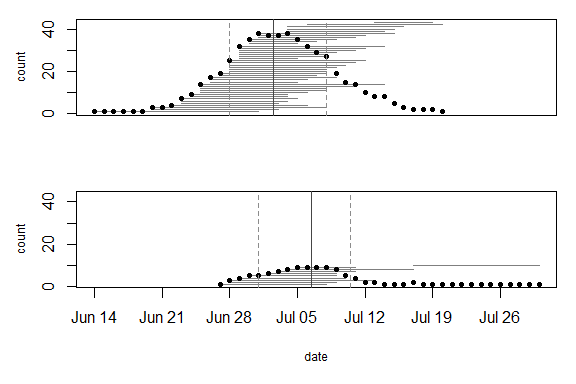Alternatively, include other columns in the makeScene argument otherCols, and specify those column names when plotting and doing other analysis:

ech2012b <- makeScene(ech2012, startCol = "firstDay", endCol = "lastDay",
xCol = "eCoord", yCol = "nCoord", idCol = "tag",
otherCols = "pop")

par(mar = c(3,4,1,1), oma = c(2,0,0,0))
plotScene(ech2012b, colorBy = 'pop')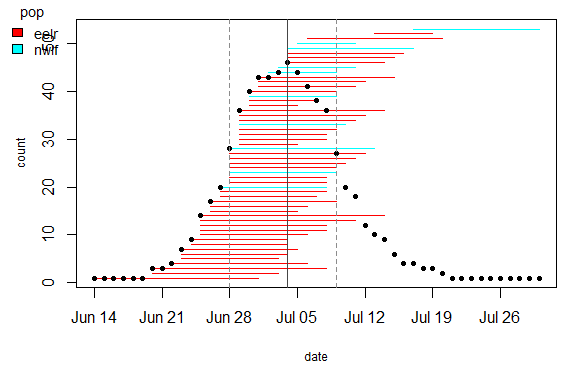plotScene(ech2012b, colorBy = 'pop', sortBy = c('pop','start','end')) # specify the stacking order of segments in the mating schedule using sortBy, listing the column names to sort by in descending levels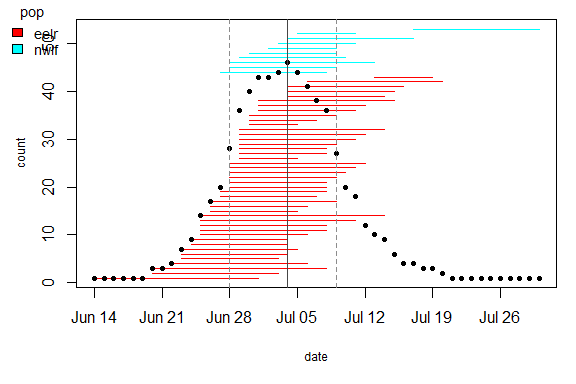# Multi-year scenes

Here we investigate the dynamics of a population’s mating scene over multiple seasons. Multi-year scenes must be formatted as lists, with each list element representing one mating scene (or one year). If we had a multi-year dataset, function makeScene would make it into a multi-year scene automatically using the argument multiYear = TRUE. Alternatively, we can simulate several mating scenes and combine them in a list by hand, as in the example below.

simScene1 <- simulateScene(size = nrow(eelr), meanSD = eSum$meanSD, sdSD = eSum$sdSD, meanDur = eSum$meanDur, sdDur = eSum$sdDur, xRange = c(eSum$minX, eSum$maxX),
yRange = c(eSum$minY, eSum$maxY))
simScene2<- simulateScene(size = 1.5*nrow(eelr), meanSD = eSum$meanSD + 365, sdSD = eSum$sdSD, meanDur = eSum$meanDur, sdDur = eSum$sdDur, xRange = c(eSum$minX, eSum$maxX),
yRange = c(eSum$minY, eSum$maxY))
## Warning in matrix(rnorm(2 * n), 2, n, byrow = FALSE): data length  is not a
## sub-multiple or multiple of the number of rows 
simScene3 <- simulateScene(size = 0.8*nrow(eelr), meanSD = eSum$meanSD + 730, sdSD = eSum$sdSD, meanDur = eSum$meanDur, sdDur = eSum$sdDur, xRange = c(eSum$minX, eSum$maxX),
yRange = c(eSum$minY, eSum$maxY))

multiYearScene <- list('2012' = simScene1,'2013' = simScene2, '2014' = simScene3)

All of the different analysis and plotting methods can be applied directly to multi-year scenes. We can make multi-panel plots of the matingScene over years. The plot limits are consistent across years, making it easier to compare differences.

plotScene(multiYearScene,sub = c(1,6,12,18,13,24,55,45,60), label.cex = 0.8)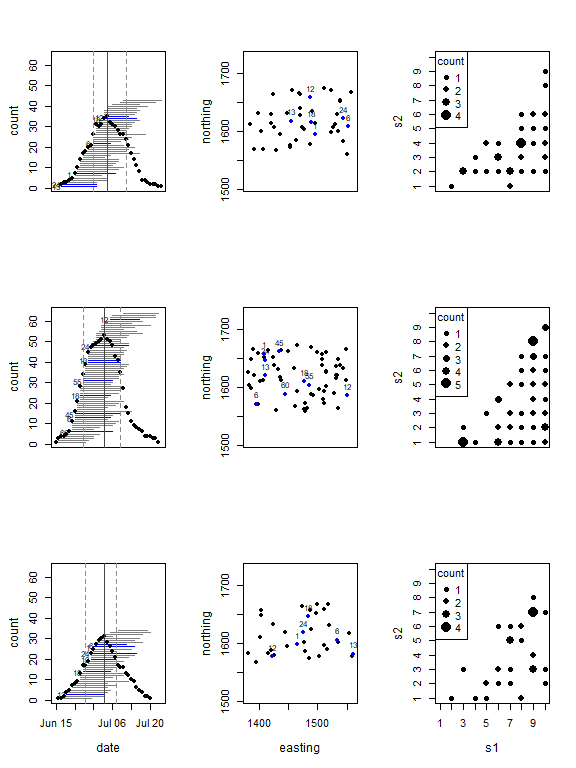We can also combine mating dimensions for multi-year plots using plot3DScene.

par(mfrow = c(3,1), mar = c(1,5,1,1), oma = c(4,4,4,0))
plot3DScene(multiYearScene, pt.cex = 1.2, sub = c(1,6,12,18,13,24,55,45,60))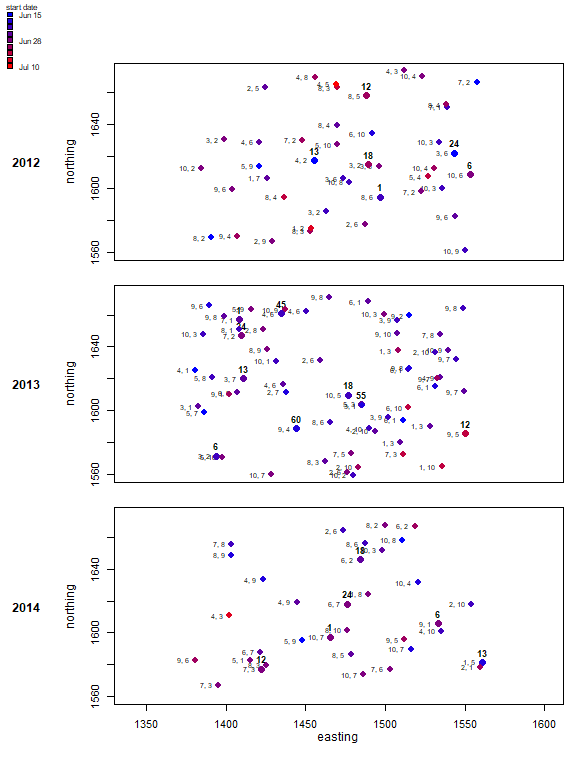The functions synchrony, proximity, and compatibility also work on multi-year scenes, returning a list of potentials objects for each year.

syncMulti <- synchrony(multiYearScene, method = 'augs')
proxMulti <- proximity(multiYearScene, method = 'maxPropSqrd')
compatMulti <- compatibility(multiYearScene, method = 'si_echinacea')

str(syncMulti) # a list of lists
## List of 3
##  $2012:List of 3 ## ..$ pop : num 0.558
##   ..$ind :'data.frame': 43 obs. of 2 variables: ## .. ..$ id       : int [1:43] 1 2 3 4 5 6 7 8 9 10 ...
##   .. ..$synchrony: num [1:43] 0.552 0.463 0.443 0.549 0.304 ... ## ..$ pair: num [1:43, 1:43] 1 1 0.25 0.357 0.692 ...
##   .. ..- attr(*, "idOrder")= int [1:43] 1 2 3 4 5 6 7 8 9 10 ...
##   ..- attr(*, "t")= logi TRUE
##   ..- attr(*, "s")= logi FALSE
##   ..- attr(*, "c")= logi FALSE
##  $2013:List of 3 ## ..$ pop : num 0.605
##   ..$ind :'data.frame': 64 obs. of 2 variables: ## .. ..$ id       : int [1:64] 1 2 3 4 5 6 7 8 9 10 ...
##   .. ..$synchrony: num [1:64] 0.73 0.735 0.72 0.731 0.674 ... ## ..$ pair: num [1:64, 1:64] 1 0.923 0.857 0.833 0.765 ...
##   .. ..- attr(*, "idOrder")= int [1:64] 1 2 3 4 5 6 7 8 9 10 ...
##   ..- attr(*, "t")= logi TRUE
##   ..- attr(*, "s")= logi FALSE
##   ..- attr(*, "c")= logi FALSE
##  $2014:List of 3 ## ..$ pop : num 0.599
##   ..$ind :'data.frame': 34 obs. of 2 variables: ## .. ..$ id       : int [1:34] 1 2 3 4 5 6 7 8 9 10 ...
##   .. ..$synchrony: num [1:34] 0.602 0.688 0.253 0.595 0.717 ... ## ..$ pair: num [1:34, 1:34] 1 0.857 0.583 0.7 0.667 ...
##   .. ..- attr(*, "idOrder")= int [1:34] 1 2 3 4 5 6 7 8 9 10 ...
##   ..- attr(*, "t")= logi TRUE
##   ..- attr(*, "s")= logi FALSE
##   ..- attr(*, "c")= logi FALSE

The functions plotPotential and plot3DPotential will then work on multi-year potential objects. For example, we can visualize synchrony over multiple years. Note that these functions will try to select the same sample of individuals across years, so if there is a year when few individuals in that sample are participating in the mating scene, there will be fewer individuals displayed in that year’s heatmap and network diagram.

par(mfrow = c(3,3))
plotPotential(syncMulti, sub.ids = c(1,6,12,18,13,24,55,44,60))
##  "synchrony"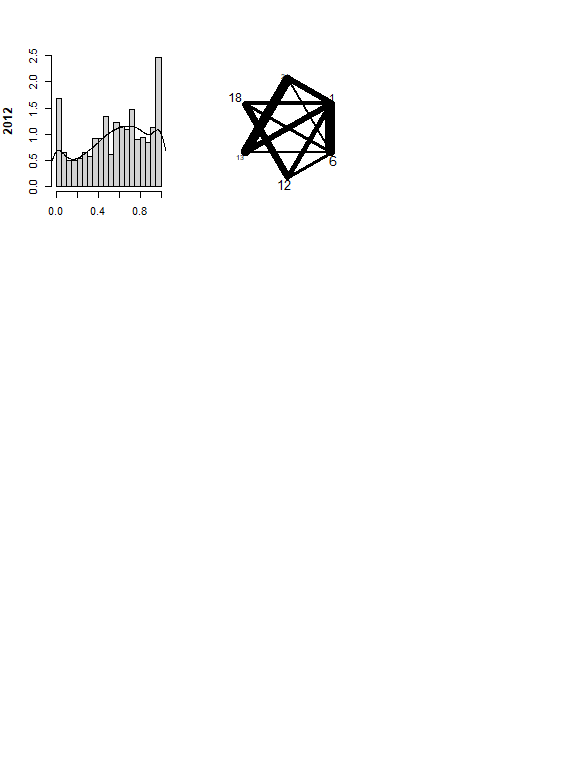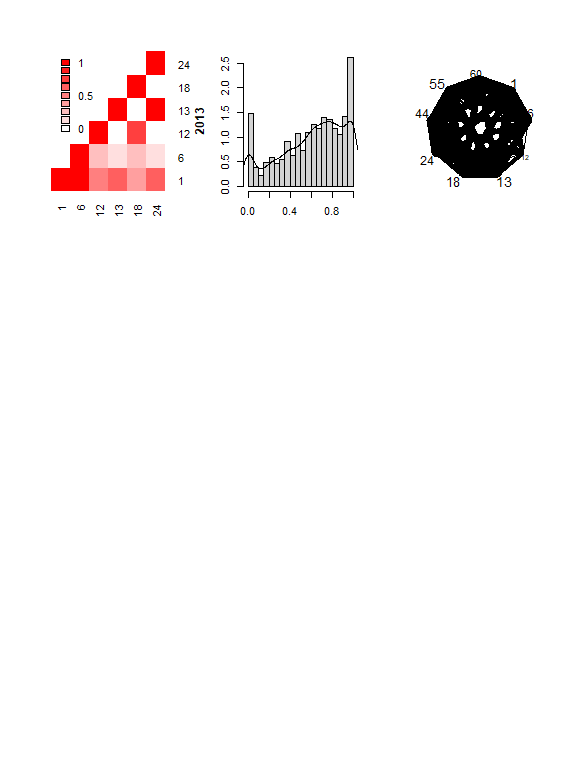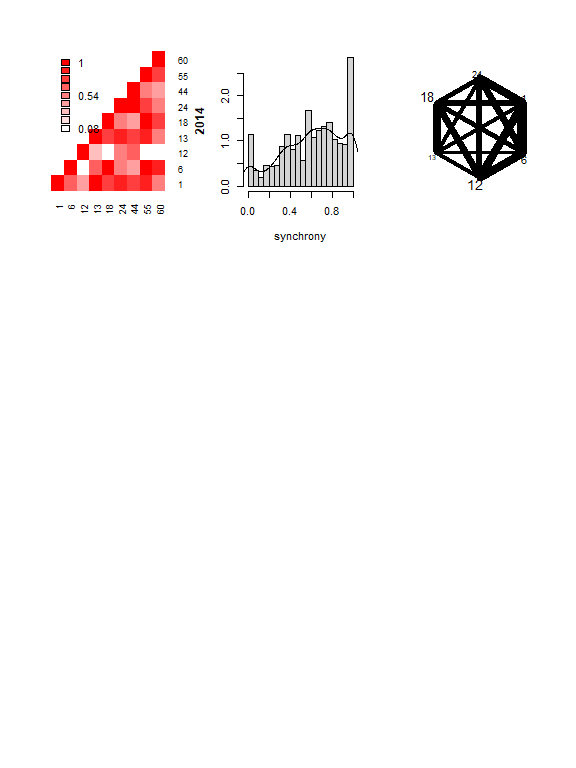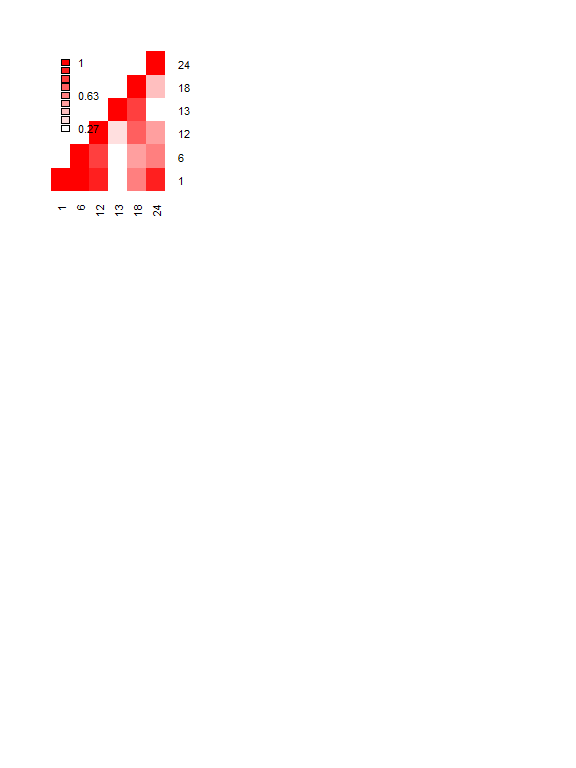And, like before, we can visualize combinations of synchrony, proximity, and compatibility over multiple years.

par(mfrow = c(3,1), mar = c(1,5,1,1), oma = c(4,4,4,0))
plot3DPotential(list(syncMulti, proxMulti, compatMulti), subject = 'ind', pt.cex = 1, sub.ids = c(1,6,12,18,13,24,55,45,60))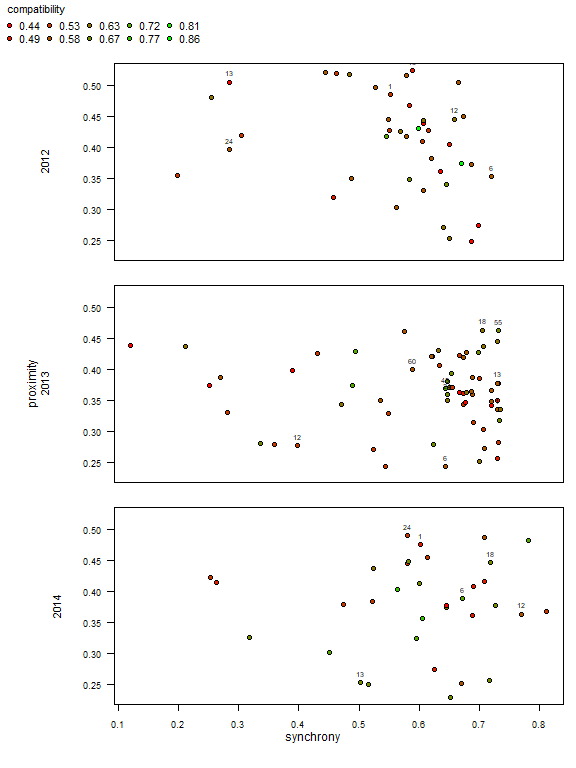Reset parameters and options.

par(oldpar)

## Conclusion

We find mateable useful and hope you do too. It can get better, so we are improving it. A development version of mateable is available via gitHub. We welcome user suggestions for improvements. Please submit bugs and feature requests to the mateable development page on github or contact Stuart directly.